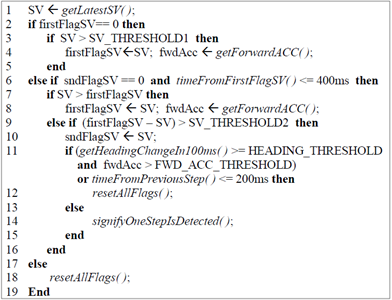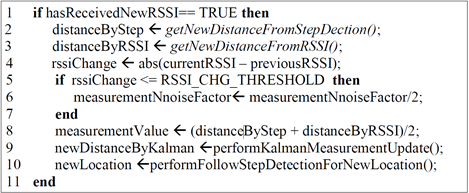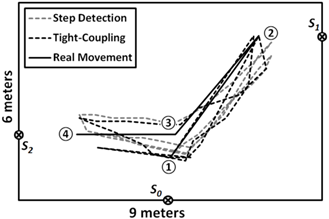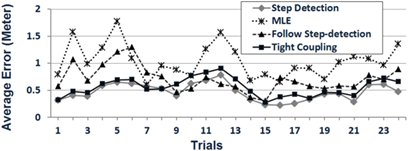Hello，everyone！

# 1. 前言

• 监测居家老年人的日常生活活动有助于发现新的或恶化的健康状况。然而，现有的室内位置跟踪系统的准确性仍不令人满意。因此，此研究目的是开发一个定位系统，能够识别患者在家庭环境中的实时位置。

• 实验结果表明，系统平均估计误差为0.47米。

# 2. 研究背景

• 由于实时家庭医疗远程监护的成本较高，因此系统改进的主要考虑因素包括更低的成本、更少的干扰以及更高的移动性、可部署性和可移植性。此类系统包括监测用户的生命体征、安全和位置跟踪。

• 为降低系统成本，论文提出了一个客户端实时室内位置跟踪系统，该系统由一个用户代理模块(基于iPhone 5)和一些低成本传感器组成。

• 系统的基本要求：在95%的置信水平下，识别患者在家庭环境中的实时位置，最大估计误差为2米。

# 4. 系统设计与实现

## 4.1 步长检测算法• 基于经验，定义两个阈值，SV_THRESHOLD1=1.07，
• 如果在200毫秒内检测到两步，则第二步被认为存在问题，因此舍弃。

## 4.2 卡尔曼滤波器

• 卡尔曼滤波器用于估计目标到BLE间的距离，表示为 d i , k d_{i,k} i i 为BLE序号， k k 为时刻。

• 当检测一步时，根据经验，设置0-0.13m作为过程噪声w_(i,k)。过程噪声的协方差 Q i = E [ w i , k , w i , k T ] Q_{i}=E\left[w_{i, k}, w_{i, k}^{T}\right] E [ . ] E[.] 为期望；测量噪声 v i , k v_{i,k} 等于转换距离乘以噪声因子0.6。时间更新方程和测量更新方程为：
d i , k − = d i , k − 1 + d i , c h g + w i , k d_{i, k}^{-}=d_{i, k-1}+d_{i, c h g}+w_{i, k}
d i , k = d i , k − + k i , k ( d i , m e a − d i , k − ) d_{i, k}=d_{i, k}^{-}+k_{i, k}\left(d_{i, m e a}-d_{i, k}^{-}\right)
其中，其中， d i , c h g d_{i,chg} 指在用户代理接收到RSSI更新之间的时间段内，目标与传感器i之间的距离变化； d i , m e a d_{i,mea} 为从传感器 i i 接收到的RSSI测量值转换而来的距离。

## 4.3 估计优化机制σ x , y = ( x − x s ) 2 + ( y − y s ) 2 \sigma_{x, y}=\sqrt{\left(x-x_{s}\right)^{2}+\left(y-y_{s}\right)^{2}}

# 5. 实验评估

## 5.1 实验设置## 5.2 性能评估03-03348607-049060
05-296058
08-229195
11-015554
05-223328
06-285852
12-261万+
06-071571
10-018万+
06-174718
06-306947
10-167640
01-203万+
05-154万+
06-124万+
04-237509
10-318791
11-061万+